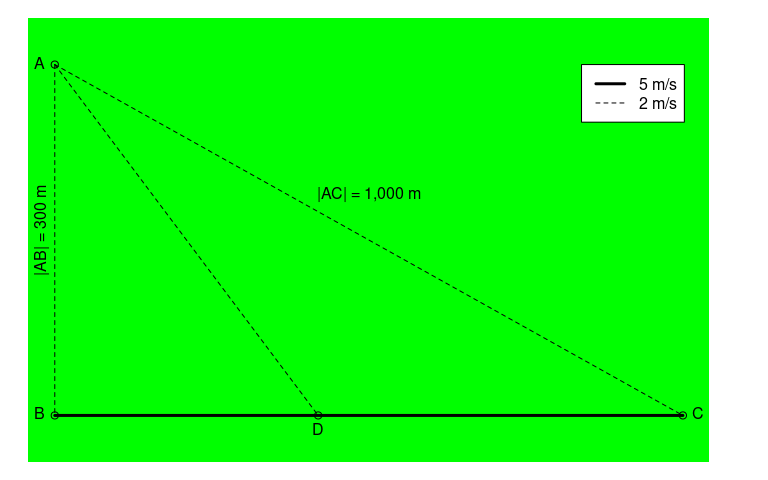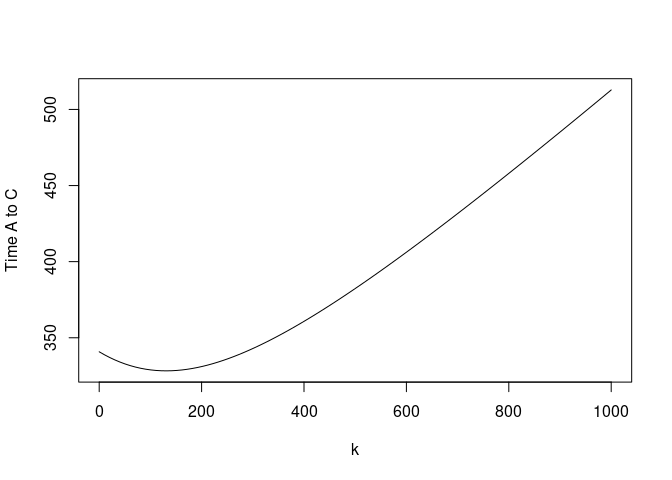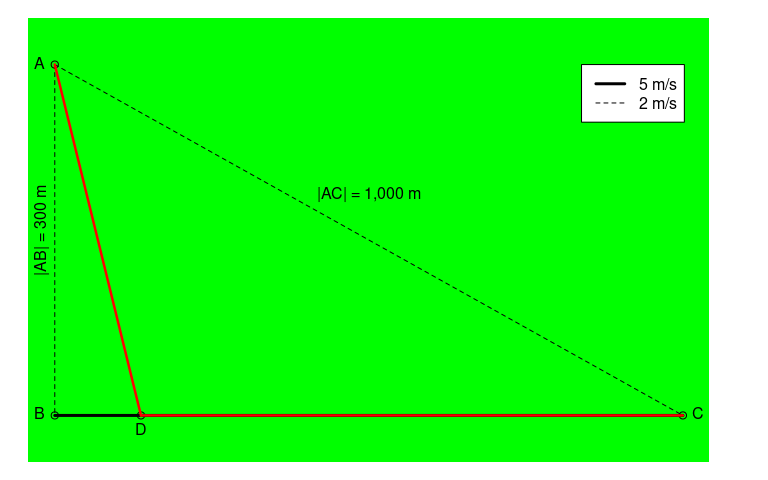Fastest route through the forest

library(caracas)

A problem was posted by the Danish newspaper, Ingeniøren, and it goes like this:

You are in the middle of a dense forest located at $$A$$. You need to get to $$C$$ in the fastest way possible, and you can only change direction once. You can walk directly via $$AB$$ to the dedicated walking path $$BC$$ where you can walk fast, you can take the direct path through the forest ($$AC$$) where you have to walk slower, or cross through the forest to the dedicated walking path ($$AD$$ and then $$DC$$).Information given

• Distances
• $$|AB| =$$ 300 m
• $$|AC| =$$ 1,000 m
• Velocities
• $$v_{AB} = 2$$ m/s
• $$v_{AD} = 2$$ m/s
• $$v_{AC} = 2$$ m/s
• $$v_{BC} = 5$$ m/s

Length of line segments

We parameterise with $$k = |BD|$$, the distance between $$B$$ and $$D$$. That is, how much to walk on fast walking path before crossing into the forest.

Formulating using caracas:

So for a distance of $$|AD|$$, you travel by 5 m/s, and then for a distance of $$- k + 100 \sqrt{91}$$ you travel by 2 m/s. Thus it takes $$\frac{\sqrt{k^{2} + 90000}}{2}$$ to travel $$AD$$ and $$- \frac{k}{5} + 20 \sqrt{91}$$ to travel $$DC$$.

The question is: What is the fastest way to get from $$A$$ to $$C$$?

First, the total duration of the route is:It looks like a minimum around $$k = 150$$.

We find the analytical solution by first finding critical points:

The type of the critical point is found by considering the Hessian:

Thus the critical point is indeed a minimum as suggested by the plot.

The fastest route is thus obtained for $k = \frac{200 \sqrt{21}}{7} \approx 130.93 .$ It has a length of (in meters)

$\frac{300 \sqrt{21}}{7} + 100 \sqrt{91} \approx 1150.34$ and takes (in seconds)

$30 \sqrt{21} + 20 \sqrt{91} \approx 328.27$

The best route can be illustrated, too: